Search IntMath
Close

450+ Math Lessons written by Math Professors and Teachers

5 Million+ Students Helped Each Year

1200+ Articles Written by Math Educators and Enthusiasts

Simplifying and Teaching Math for Over 23 Years

# 5. Solving Trigonometric Equations

by M. Bourne

Trigonometric equations can be solved using the algebraic methods and trigonometric identities and values discussed in earlier sections. You may wish to go back and have a look at Trigonometric Functions of Any Angle, where we see the background to the following solutions.

A painless way to solve these is using a graph. Where the graph cuts the x-axis, that's where you'll find your solutions (the x-values that "work"). Graphs also help you to understand why sometimes there is one answer, and sometimes many answers. I use Scientific Notebook or similar math software to graph the functions for me.

You can use this Online Graphing Calculator to solve the following equations (or check your solutions) .

### Example 1

Solve the equation 2 cos θ − 1 = 0 for 0 ≤ θ < 2π.

Rearranging the above equation, we get:

cos theta=1/2

We know the following :

Since

cos (pi/3) = 1/2

and cos θ is positive in the first and fourth quadrants, we have:

theta=pi/3

or

theta=2pi-pi/3=(5pi)/3

So theta=pi/3 or theta=(5pi)/3

### Revision Tip

Getting lost in this section? See the background at Trigonometric Functions of Any Angle

Graphically solve the equation

2 cos2x − sin x − 1 = 0

such that 0 ≤ θ < 2π.

Using graphing software, we draw the curve of y = 2 cos2x − sin x − 1 in the region 0 ≤ θ < 2π. Wherever the curve cuts the x-axis will be the solution for our equation.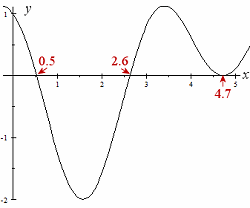We see from the graph that the solutions are approximately:

x = 0.5
x = 2.6
x
= 4.7

For more accurate solutions, we would just zoom in on the graph.

In this next graph, I have zoomed in to the second root (the one near x = 2.6). We can see that this root is x = 2.618 correct to 3 decimal places.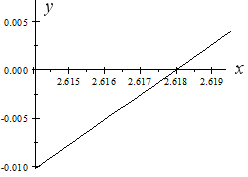We could continue to zoom in as close as we like to get the accuracy that we require.

Continues below

## Solving Equations Involving Multiples of θ

### Example 3

Solve the equation sin 2θ = 0.8 for 0 ≤ θ < 2π.

If the problem involved θ only, we would expect 2 solutions; one in the first quadrant and one in the second quadrant.

But here our problem involves 2θ, so we have to double the domain (θ values) to account for all possible solutions.

We proceed as follows:

We solve

sin 2θ = 0.8 for 0 ≤ 2θ < 4π.

The reference angle is

α = arcsin 0.8 = 0.9273

So the values for 2θ will be in quadrants I, II, V, VI.

2θ = 0.9273, or  π − 0.9273, or   2π + 0.9273, or 3π − 0.9273

That is

2θ = 0.9273, 2.2143, 7.2105, 8.4975

But we need values for θ, not 2θ, so we divide throughout by 2:

θ = 0.4637, 1.1072, 3.6053, 4.2488

Are our answers correct? As usual, we will check by graphing the original expression: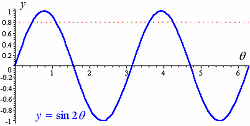We can see from the graph that our 4 values are reasonable, since these are the only 4 values that satisfy sin 2θ = 0.8.

Note: We can always check our solutions with calculator, but it is easy to "miss out" on some of the required values if we only use calculator.

### Example 4

Solve the equation

cos^2theta=1/16

for 0 ≤ θ < 2π.

Solving for cos θ gives us:

cos theta=+-1/4

If cos alpha=1/4, then the reference angle is α = 1.3181.

So for cos theta=1/4, we have θ in the first and 4th quadrants. So

θ = 1.3181 or 4.9651

For cos theta=-1/4, we have θ in the 2nd and 3rd quadrants. So

θ = 1.8235 or 4.4597

So  θ= 1.3181, 1.8235,  4.4597  or 4.9651 radians.

We can see from the graph of y=cos^2theta-1/16 that our answer is correct: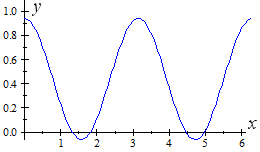### Example 5

Solve the equation

6 sin2θ − sin θ − 1 = 0

for 0 ≤ θ < 2π.

Factoring the LHS:

6 sin^2θ − sin θ − 1 = 0

(2 sin θ − 1)(3 sin θ + 1) = 0

So either

2 sin θ − 1 = 0

sin θ = 1/2

θ will be in 1st and 2nd quadrants.

θ = 0.52360, 2.6180 (that is, pi/6 or (5pi)/6)

OR

3 sin θ + 1 = 0

sin θ = − 1/3

θ will be in 3rd and 4th quadrants.

θ = 3. 4814, 5. 9433

Checking our solution on a graph: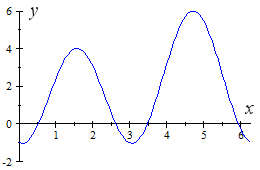So θ = 0.52360, 2.6180, 3. 4814, 5. 9433

### Example 6

Solve the equation

cos{:x/2:}=1+cos\ x

for 0 ≤ θ < 2π.

Earlier we learned that cos(x/2)=+-sqrt((1+cos x)/2), so we have:

+-sqrt((1+cos x)/2)= 1 + cos x

Squaring both sides gives:

(1+cos x)/2=(1+cos x)^2

(1+cos x)/2=1+2\ cos x+cos^2x

1+cos x=2+4\ cos x+2\ cos^2x

So we have:

2\ cos^2 \ x + 3\ cos x + 1 = 0

(2\ co\s x + 1)(cos x + 1) = 0

Solving, we get

cos x = − 0.5 or cos x = − 1

Now cos x=-1/2 gives x=(2pi)/3,(4pi)/3.

However, on checking in the original equation, we note that

"LHS"=cos((4pi)/3xx1/2) =cos((2pi)/3) =-1/2

but

"RHS"=1+cos (4pi)/3=+1/2

So the only solution for this part is x=(2pi)/3.

Also, cos x=-1 gives x = pi.

So the solutions for the equation are x=(2pi)/3or pi.

A check of the graph of y=cos x/2-1-cos x confirms these results: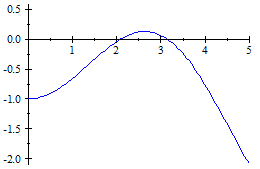(2π/3 ≈ 2.0944 and π ≈ 3.14).

### Example 7

Solve the equation

tan 2θ − cot 2θ = 0

for 0 ≤ θ < 2π.

cot 2theta= 1/(tan 2 theta), so we have:

tan 2 theta-1/(tan 2 theta)=0

tan^2 2θ = 1

tan 2θ = ± 1

Since 0 ≤ θ < 2π, we need to consider values of 2theta such that 0 ≤ 2θ < 4π. Hence, solving the above equation, we have:

2 theta=pi/4,(3pi)/4,(5pi)/4(7pi)/4, (9pi)/4,(11pi)/4, (13pi)/4,(15pi)/4

Dividing throughout by 2 gives us the full set of solutions in the required domain, 0 <= theta <2pi:

theta=pi/8,(3pi)/8, (5pi)/8,(7pi)/8, (9pi)/8,(11pi)/8, (13pi)/8,(15pi)/8

## Exercises

Note 1: "Analytically" means use the methods and formulas from previous sections. It means don't just use a graph to solve it.

Note 2: However, I always use a graph to check my analytical work. I can see immediately if some error has occurred. I encourage you to do the same!

1. Solve the trigonometric equation analytically

4 tan x − sec2 x = 0 (for 0 ≤ x < 2π)

4\ tan x− sec^2x= 0

Writing this in terms of sin x and cos x only:

4(sin x)/(cos x)-1/(cos^2x)=0

Multiplying throughout by cos x:

4\ sin x\ cos x=1

Dividing both sides by 2:

2\ sin x\ cos x=1/2

Recognizing the LHS is sin 2x, from before:

sin 2x,=0.5

In 0 ≤ x < 2π, we need to find values of 2x such that 0 ≤ 2x < 4π. (Twice the original domain.)

So the values for 2x are:

2x=pi/6,(5pi)/6,(13pi)/6,(17pi)/6

Dividing throughout by 2 gives our required values for x:

x=pi/12,(5pi)/12,(13pi)/12,(17pi)/12

or, in decimal form:

x = 0.2618, 1.309, 3.403, 4.451

2. Solve the trigonometric equation analytically for 0 ≤ x < 2π:

sin 2x cos x − cos 2x sin x = 0

We recognise the left hand side to be in the form:

sin(a − b) =  sin a cos b − cos a sin b,

where a = 2x and b =x.

So

sin 2x\ cos x − cos 2x\ sin x

= sin(2x − x)

= sin x

Now, we know the solutions of sin x = 0 to be:

x = 0, π.

[Why?]

3. Solve the given trigonometric equation analytically and by graphical method (for 0 ≤ x < 2π):

sin 4x − cos 2x = 0

sin 4x − cos 2x = 0

2sin 2x cos 2x − cos 2x = 0

Factoring gives:

cos 2x (2 sin 2x − 1) = 0

EITHER

cos 2x= 0

2x=pi/2,(3pi)/2,(5pi)/2,(7pi)/2

x=pi/4,(3pi)/4,(5pi)/4,(7pi)/4

OR

sin 2x= 1/2

2x=pi/6,(5pi)/6,(13pi)/6,(17pi)/6

x=pi/12,(5pi)/12,(13pi)/12,(17pi)/12

Or, in decimal form:

x= 0.26, 0.79,  1.31, 2.36,  3.40, 3.93,  4.45, 5.50.

The graph of y = sin 4x− cos 2x is as follows. We can see from where the graph cuts the x-axis that our answers are reasonable.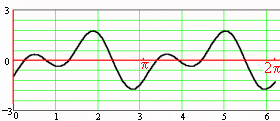## Problem SolverThis tool combines the power of mathematical computation engine that excels at solving mathematical formulas with the power of GPT large language models to parse and generate natural language. This creates math problem solver thats more accurate than ChatGPT, more flexible than a calculator, and faster answers than a human tutor. Learn More.